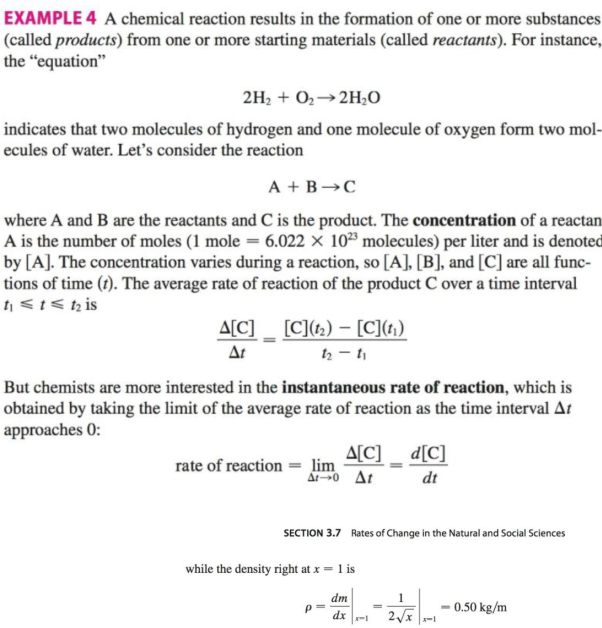Chapter 3.7, Problem 24E

Chapter
Section
Textbook Problem

If, in Example 4, one molecule of the product C is formed from one molecule of the reactant A and one molecule of the reactant B, and the initial concentrations of A and B have a common value [A] = [B] = a moles/ L, then[C] = a2kt/(akt + 1)where k is a constant.(a) Find the rate of reaction at time t.(b) Show that if x = [C], then d x d t = k ( a − x ) 2 c) What happens to the concentration as t → ∞?(d) What happens to the rate of reaction as t →4 ∞?(e) What do the results of parts (c) and (d) mean in practical terms?EXAMPLE 4(a)

To determine

To find: The rate of reaction at time t.

Explanation

Given:

The expression is given as below.

[C]=a2ktakt+1 (1)

Here, the variable [C] is the product, and a is the initial concentration of [A]=[B] in moles/L.

Calculation:

Differentiate equation (1) with respect t.

d[C]dt=[(akt+1)(a2k)](a2kt)(ak)(akt+

(b)

To determine

To show: The equation dxdt=k(ax)2, where [C]=x.

(c)

To determine

To show: The concentration if time t tends to .

(d)

To determine

To find: The rate of reaction if time t tends to .

(e)

To determine

To compare: The practical means of part (c) and (d).

Still sussing out bartleby?

Check out a sample textbook solution.

See a sample solution

The Solution to Your Study Problems

Bartleby provides explanations to thousands of textbook problems written by our experts, many with advanced degrees!

Get Started

Solve the equations in Exercises 126. 4xx313x4x31x31=0

Finite Mathematics and Applied Calculus (MindTap Course List)